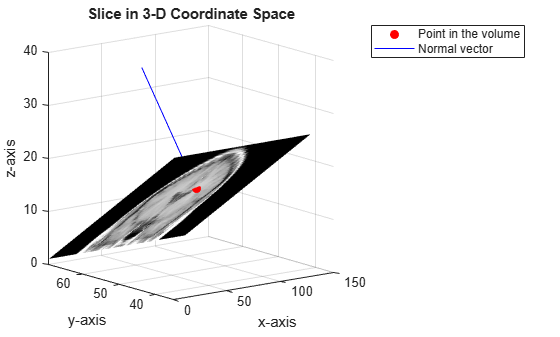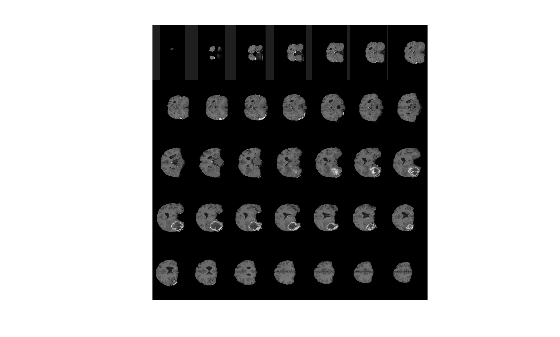# obliqueslice

Extract oblique slice from 3-D volumetric data

## Syntax

``B = obliqueslice(V,point,normal)``
``B = obliqueslice(___,Name,Value)``
``[B,x,y,z] = obliqueslice(___)``

## Description

example

````B = obliqueslice(V,point,normal)` extracts 2-D oblique slice from a 3-D volumetric data `V`. The slice is extracted with reference to a given point on the volume and a normal vector. The slicing plane is perpendicular to the normal vector and passes through the specified point.For information about how the slice is extracted with respect to the given point and the normal, see Oblique Slicing. The orientation of the extracted slice in image plane depend on its position in the 3-D coordinate space. For more information, see Orientation of Slice in Image Plane.```

example

````B = obliqueslice(___,Name,Value)` specifies options using one or more name-value arguments in addition to the input arguments in the previous syntax.```

example

````[B,x,y,z] = obliqueslice(___)` also returns the 3-D Cartesian coordinates of the extracted slice in the input volume.```

## Examples

collapse all

Load a 3-D volumetric data into the workspace.

`load mri`

Remove singleton dimensions by using the `squeeze` function.

`V = squeeze(D);`

Display horizontal slices of the data by using the `montage` function.

```figure montage(V,map,'Size',[3 9]);```Specify a point in the volume for the slice to pass through.

`point = [73 50 15.5];`

Specify the normal vector to a plane in 3-D coordinate space.

`normal = [0 15 20];`

Extract a slice from the volumetric data. The slice is perpendicular to the normal vector and passes through the specified point.

`[B,x,y,z] = obliqueslice(V,point,normal);`

Display the extracted slice in the 3-D coordinate space.

```fig = figure; axes1 = axes('Parent',fig,'Position',[0.13 0.4 0.37 0.5]); surf(x,y,z,B,'EdgeColor','None','HandleVisibility','off','Parent',axes1); grid on view([-62.6 13.8]) colormap(gray) xlabel('x-axis') ylabel('y-axis'); zlabel('z-axis'); title('Position of Slice in 3-D Coordinate Space')```

Plot the point and the normal vector.

```hold on plot3(point(1),point(2),point(3),'or','MarkerFaceColor','r'); plot3(normal(1),normal(2),normal(3),'ob','MarkerFaceColor','b'); hold off legend('Point in the volume','Normal vector','Position',[0.15 0.2 0.3 0.08])```

Display the extracted slice in the image plane.

```axes2 = axes('Parent',fig,'Position',[0.59 0.52 0.33 0.6]); imshow(B,[],'Parent',axes2) title('Output Slice in Image Plane')```Load a 3-D volumetric data into the workspace.

```s = load(fullfile(toolboxdir('images'),'imdata','BrainMRILabeled','images','vol_001.mat')); V = s.vol;```

Display horizontal slices of the data by using the `montage` function.

```figure montage(V,'Indices',12:118,'Size',[8 12],'DisplayRange',[]);```Specify the normal vector to a plane in 3-D coordinate space.

`normal = [10 0 5];`

Use a `for` loop to extract multiple slices along the direction of the normal vector. In each iteration, specify a point that the slice has to pass through. Set the output size to `'Full'` and the fill value for padding pixels to 255.

```cnt = 1; for xslice = 10:5:180 point{cnt} = [xslice 150 80]; [B(:,:,cnt),x(:,:,cnt),y(:,:,cnt),z(:,:,cnt)] = obliqueslice(V,point{cnt},normal,... 'OutputSize','Full','FillValues',255); cnt = cnt+1; end ```

Display the extracted slices. The extracted slices are perpendicular to the normal vector and passes through the specified point.

```figure for slice = 1:size(B,3) subplot('Position',[0.11 0.36 0.38 0.5]) surf(x(:,:,slice),y(:,:,slice),z(:,:,slice),B(:,:,slice), ... 'EdgeColor','None','HandleVisibility','off'); grid on view([-24 12]) colormap(gray) xlabel('x-axis') ylabel('y-axis'); zlabel('z-axis'); zlim([0 155]); ylim([0 250]); xlim([0 250]); title('Position of Slice in 3-D Coordinate Space') % Plot the point and the normal vector. hold on plot3(point{slice}(1),point{slice}(2),point{slice}(3),'or','MarkerFaceColor','r'); plot3(normal(1),normal(2),normal(3),'ob','MarkerFaceColor','b'); legend('Point in the volume','Normal vector','Position',[0.1 0.12 0.3 0.08]) hold off % Display the extracted slice. subplot('Position',[0.6 0.37 0.34 0.49]) imshow(B(:,:,slice),[]) title('Output Slice in Image Plane') pause(0.5); end```Display the extracted image slices by using the `montage` function.

```figure montage(B,'Size',[5 7],'DisplayRange',[]);```## Input Arguments

collapse all

Input volume, specified as a 3-D numeric or categorical array.

Data Types: `single` | `double` | `int8` | `int16` | `int32` | `uint8` | `uint16` | `uint32` | `logical` | `categorical`

Point in the volume, specified as a 3-element row vector of form [px py pz].

Data Types: `single` | `double` | `int8` | `int16` | `int32` | `int64` | `uint8` | `uint16` | `uint32` | `uint64`

Normal vector, specified as a 3-element row vector of form [`a` `b` `c`].

To extract an orthogonal slice, you can set the normal vector to one of these values:

• [1 0 0] — Extract slice in the yz-plane.

• [0 1 0] — Extract slice in the xz-plane.

• [0 0 1] — Extract slice in the xy-plane.

Data Types: `single` | `double` | `int8` | `int16` | `int32` | `int64` | `uint8` | `uint16` | `uint32` | `uint64`

### Name-Value Pair Arguments

Specify optional comma-separated pairs of `Name,Value` arguments. `Name` is the argument name and `Value` is the corresponding value. `Name` must appear inside quotes. You can specify several name and value pair arguments in any order as `Name1,Value1,...,NameN,ValueN`.

Example: `obliqueslice(V,point,normal,'OutputSize','Full')`

Interpolation method, specified as the comma-separated pair consisting of `'Method'` and any one of these values:

• `'linear'` — linear interpolation

• `'nearest'` — nearest neighbor interpolation

If `V` is numeric, the interpolation method defaults to `'linear'` but can also be specified as `'nearest'`. If `V` is categorical, the interpolation method must be `'nearest'`.

Data Types: `char` | `string`

Size of output image, specified as the comma-separated pair consisting of `'OutputSize'` and any of these values:

• `'limit'` — The size of the output image is the actual size of the 2-D slice with respect to the dimensions of input volume. If the extracted slice region is not square or rectangular, the function automatically pads the extracted slice region with extra pixels to yield a square or rectangular image.

• `'full'` — The size of the output image may not be equal to the actual size of the 2-D slice. The size of the output image is set to the maximal slice size that can be obtained from the input volume with respect to the normal vector `normal`. To resize the image, the border of the extracted 2-D slice is padded with extra rows and columns.

The fill value for the padded pixels is 0 by default. You can use the '`FillValues`' name-value pair argument to change the value.

Data Types: `char` | `string`

Fill value for padded pixels, specified as the comma-separated pair consisting of `'FillValues'` and a scalar, character vector, or `missing`.

When `V` is a numeric array, specify

When `V` is a categorical array, specify

• character vector that denotes a category in the input data. To know the categories, use the `categories` function.

• `missing`, if the category in input data is equal to `<undefined>`.

Data Types: `single` | `double` | `int8` | `int16` | `int32` | `int64` | `uint8` | `uint16` | `uint32` | `uint64` | `char`

## Output Arguments

collapse all

Output 2-D slice, returned as a numeric or categorical matrix of size m-by-n. The data type of the output slice is same as the data type of the input volume.

Data Types: `single` | `double` | `int8` | `int16` | `int32` | `uint8` | `uint16` | `uint32` | `logical` | `categorical`

x-coordinates of the output slice in 3-D volume, returned as a m-by-n matrix of the size same as the output slice.

Data Types: `single`

y-coordinates of the output slice in 3-D volume, returned as a m-by-n matrix of the size same as the output slice.

Data Types: `single`

z-coordinates of the output slice in 3-D volume, returned as a m-by-n matrix of the size same as the output slice.

Data Types: `single`

collapse all

### Oblique Slicing

Given a point (px, py, pz) and the normal vector (a, b, c), the function solves the plane equation a(x-px)+b(y-py)+c(z-pz) = 0The point (px, py, pz) lies in the volumetric data. The slicing plane is perpendicular to the normal vector and passes through the given point.

### Orientation of Slice in Image Plane

The orientation of the extracted slice in the image plane depends on its inclination angle with respect to the horizontal and vertical planes.

The slice corner that lies close to the origin of the last slice, (0, 0, P), in the volumetric data constitutes the upper-left pixel in the image plane. To fill pixel values in the image plane, start from the slice corner and read the intensity values in left-to-right, top-to-bottom scan order.### Objects

Introduced in R2020a